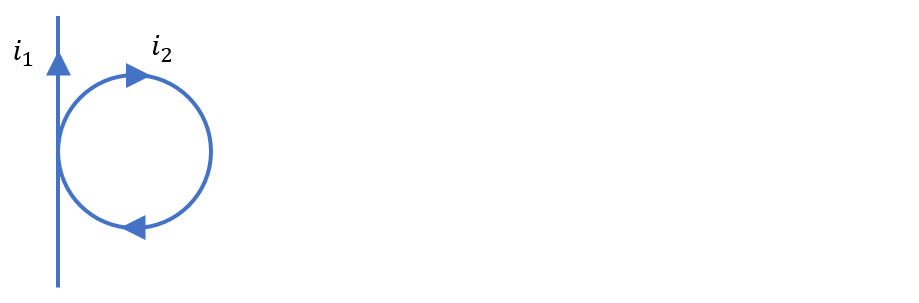# Problem: A long, straight wire carries a current of I 1 = 8.0 A. A circular loop of wire lies immediately to the right of the straight wire, with the plane of the loop parallel to the wire. The loop has a radius of R = 0.03 m and carries a current of I2 = 2.0 A. Assuming that the thickness of the wires is negligible, find the magnitude and direction of the net magnetic field at the center of the loop.

🤓 Based on our data, we think this question is relevant for Professor Schulte's class at UCF.

###### Problem Details

A long, straight wire carries a current of I 1 = 8.0 A. A circular loop of wire lies immediately to the right of the straight wire, with the plane of the loop parallel to the wire. The loop has a radius of R = 0.03 m and carries a current of I2 = 2.0 A. Assuming that the thickness of the wires is negligible, find the magnitude and direction of the net magnetic field at the center of the loop.